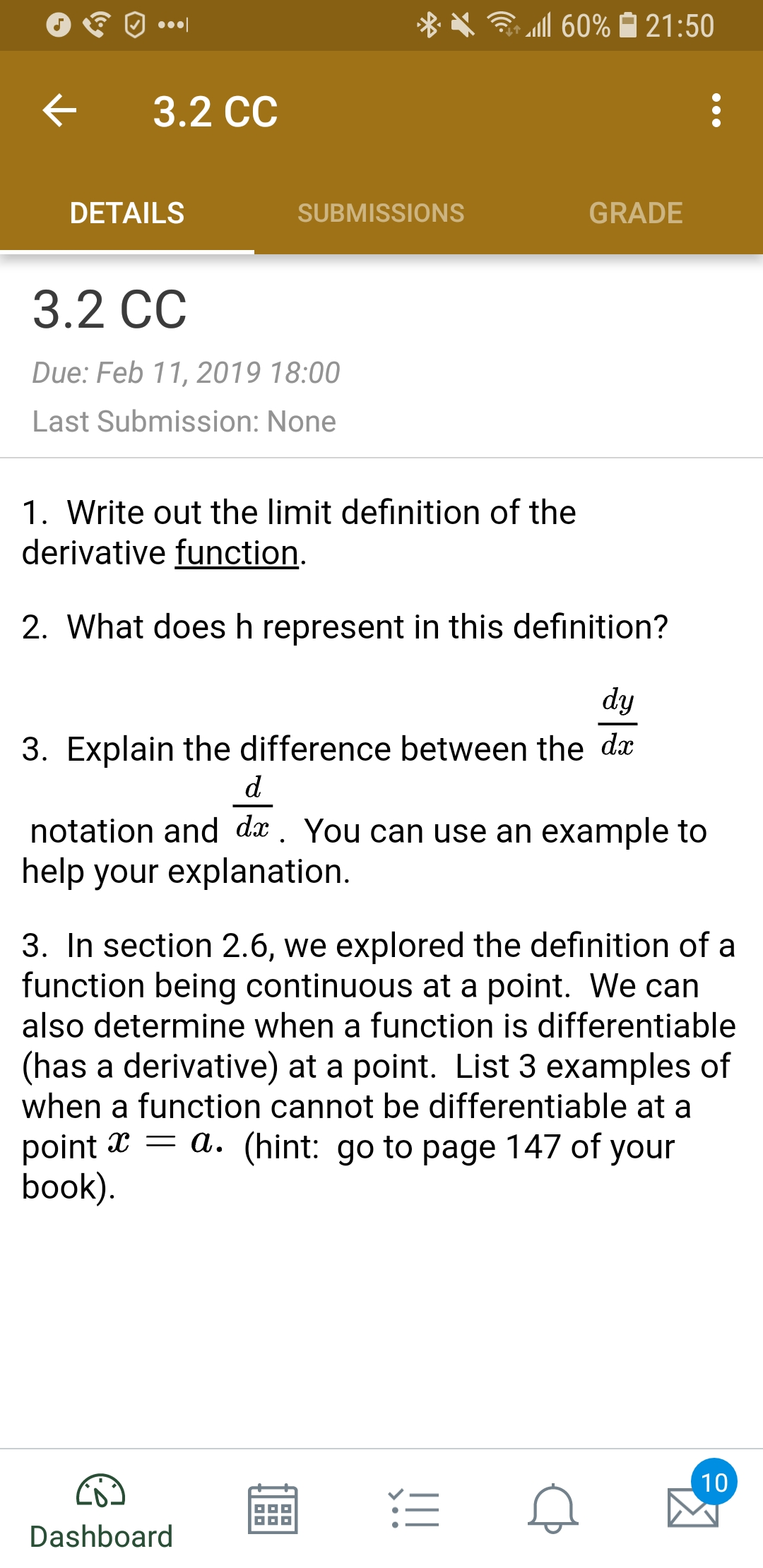# X .111 60% 21:50< 3.2 ccDETAILSSUBMISSIONSGRADE3.2 CCDue: Feb 11, 2019 18:00Last Submission: None1. Write out the limit definition of thederivative function2. What does h represent in this definition?3. Explain the difference between the dxnotation and dx. You can use an example tohelp your explanation3. In section 2.6, we explored the definition of afunction being continuous at a point. We canalso determine when a function is differentiable(has a derivative) at a point. List 3 examples ofwhen a function cannot be differentiable at apoint x - a. (hint: go to page 147 of yourbookDashboard

Question
12 views

What are three examples of when a function is not differential? (Last Question)help_outlineImage TranscriptioncloseX .111 60% 21:50 < 3.2 cc DETAILS SUBMISSIONS GRADE 3.2 CC Due: Feb 11, 2019 18:00 Last Submission: None 1. Write out the limit definition of the derivative function 2. What does h represent in this definition? 3. Explain the difference between the dx notation and dx. You can use an example to help your explanation 3. In section 2.6, we explored the definition of a function being continuous at a point. We can also determine when a function is differentiable (has a derivative) at a point. List 3 examples of when a function cannot be differentiable at a point x - a. (hint: go to page 147 of your book Dashboard fullscreen
check_circle

Step 1

For checking the differentiability, we are going ot use standard method of checking differentiablity.

If the limits in both sides are equal then function f(x) is equal then our function is Differentiable and if limits are not equal then it is not differentiable.

Step 2

Example 1) In this example we are showing that function is not differentiable at X=a.

In this example,Since the limit on both sides are different 1 and 0 . Therefore it is not differentiable.

Step 3

Example 1) In this example we are showing that function is not differentiable at X=a-h.

In this exampl...

### Want to see the full answer?

See Solution

#### Want to see this answer and more?

Solutions are written by subject experts who are available 24/7. Questions are typically answered within 1 hour.*

See Solution
*Response times may vary by subject and question.
Tagged in

### Calculus